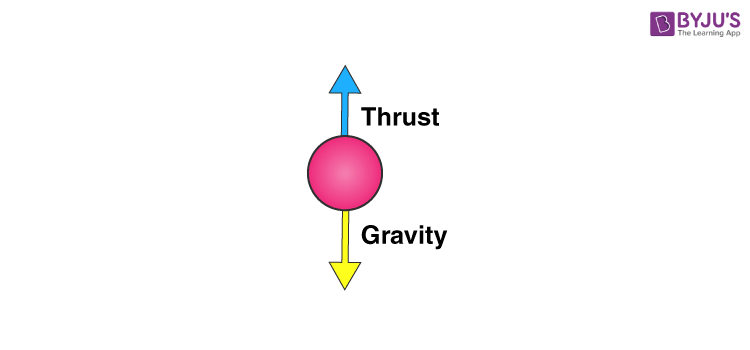# Thrust And PressureYou may have noticed that when you submerge something in water it appears to weigh less. If you have gone swimming, you feel as though your weight has reduced. This is because any fluid applies an upward force on a body submerged in it. This force is called thrust. This force is in the opposite direction to the force of gravity. Hence, it reduces the total force acting downwards due to gravity on the object and you feel as though your weight has reduced.### Pressure

Pressure is defined as the force per unit area. If a force F is applied on a surface of area A, then the pressure P is defined as:

P = $\frac {F}{A}$

As you blow up a balloon, it becomes harder to blow the balloon as it becomes bigger and bigger. This is because the air pressure inside the balloon is increasing. Pressure is expressed in N/m2 which is also called a Pascal (Pa).

In fluids this pressure is always perpendicular to the surface of an object. It is this pressure that is applied on an object submerged in water that forms the thrust force.

The pressure applied by the fluid is:

$Pressure$ = $\frac {Thrust}{Area}$

Also,

$1~Pascal ~(Pa)$ = $\frac {1~Newton~(N)}{1~Metre~square~(m^2)}$

Or

$Thrust$  = $Pressure~×~Area$

This pressure which produces a thrust on the object is the reason some objects float in water. It is also the reason why objects weigh less in water. To know why objects float or sink, see our article on the Archimedes Principle. Download BYJU’s-The Learning App to learn practically and interactively. We also have loads of fun learning videos and practice questions that you can do at your own pace. So go ahead and check it out in Byju’s.

Test your knowledge on Thrust Pressure

1. Nandana

Nice